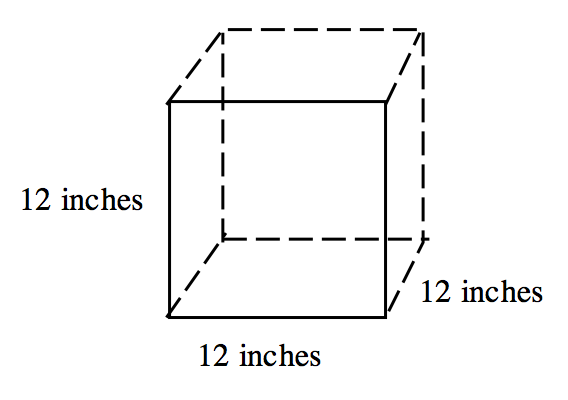### Home > INT2 > Chapter 11 > Lesson 11.2.2 > Problem11-68

11-68.

A cube has an edge length of $12$ inches.

1. Draw a diagram of the cube and calculate the volume and surface area in cubic and square inches respectively.Volume $= (12)(12)(12) = 1728$ in$^3$
Surface Area $= (6)(12)(12) = 864$ in$^2$

2. Now calculate the volume and surface area in cubic and square feet respectively.

Converting from in$^3$ to ft$^3$ means you must use a conversion factor of ($12$ in)$^3$, not just $12$ in.